## 5.41. atmost_nvalue

Origin
Constraint

$\mathrm{𝚊𝚝𝚖𝚘𝚜𝚝}_\mathrm{𝚗𝚟𝚊𝚕𝚞𝚎}\left(\mathrm{𝙽𝚅𝙰𝙻},\mathrm{𝚅𝙰𝚁𝙸𝙰𝙱𝙻𝙴𝚂}\right)$

Synonyms

$\mathrm{𝚜𝚘𝚏𝚝}_\mathrm{𝚊𝚕𝚕𝚍𝚒𝚏𝚏}_\mathrm{𝚖𝚊𝚡}_\mathrm{𝚟𝚊𝚛}$, $\mathrm{𝚜𝚘𝚏𝚝}_\mathrm{𝚊𝚕𝚕𝚍𝚒𝚏𝚏𝚎𝚛𝚎𝚗𝚝}_\mathrm{𝚖𝚊𝚡}_\mathrm{𝚟𝚊𝚛}$, $\mathrm{𝚜𝚘𝚏𝚝}_\mathrm{𝚊𝚕𝚕𝚍𝚒𝚜𝚝𝚒𝚗𝚌𝚝}_\mathrm{𝚖𝚊𝚡}_\mathrm{𝚟𝚊𝚛}$.

Arguments
 $\mathrm{𝙽𝚅𝙰𝙻}$ $\mathrm{𝚍𝚟𝚊𝚛}$ $\mathrm{𝚅𝙰𝚁𝙸𝙰𝙱𝙻𝙴𝚂}$ $\mathrm{𝚌𝚘𝚕𝚕𝚎𝚌𝚝𝚒𝚘𝚗}\left(\mathrm{𝚟𝚊𝚛}-\mathrm{𝚍𝚟𝚊𝚛}\right)$
Restrictions
 $\mathrm{𝙽𝚅𝙰𝙻}\ge \mathrm{𝚖𝚒𝚗}\left(1,|\mathrm{𝚅𝙰𝚁𝙸𝙰𝙱𝙻𝙴𝚂}|\right)$ $\mathrm{𝚛𝚎𝚚𝚞𝚒𝚛𝚎𝚍}$$\left(\mathrm{𝚅𝙰𝚁𝙸𝙰𝙱𝙻𝙴𝚂},\mathrm{𝚟𝚊𝚛}\right)$
Purpose

The number of distinct values taken by the variables of the collection $\mathrm{𝚅𝙰𝚁𝙸𝙰𝙱𝙻𝙴𝚂}$ is less than or equal to $\mathrm{𝙽𝚅𝙰𝙻}$.

Example
 $\left(4,〈3,1,3,1,6〉\right)$ $\left(3,〈3,1,3,1,6〉\right)$ $\left(1,〈3,3,3,3,3〉\right)$

The first $\mathrm{𝚊𝚝𝚖𝚘𝚜𝚝}_\mathrm{𝚗𝚟𝚊𝚕𝚞𝚎}$ constraint holds since the collection $〈3,1,3,1,6〉$ involves at most 4 distinct values (i.e., in fact 3 distinct values).

Typical
 $\mathrm{𝙽𝚅𝙰𝙻}>1$ $\mathrm{𝙽𝚅𝙰𝙻}<|\mathrm{𝚅𝙰𝚁𝙸𝙰𝙱𝙻𝙴𝚂}|$ $|\mathrm{𝚅𝙰𝚁𝙸𝙰𝙱𝙻𝙴𝚂}|>1$
Symmetries
• $\mathrm{𝙽𝚅𝙰𝙻}$ can be increased.

• Items of $\mathrm{𝚅𝙰𝚁𝙸𝙰𝙱𝙻𝙴𝚂}$ are permutable.

• All occurrences of two distinct values of $\mathrm{𝚅𝙰𝚁𝙸𝙰𝙱𝙻𝙴𝚂}.\mathrm{𝚟𝚊𝚛}$ can be swapped; all occurrences of a value of $\mathrm{𝚅𝙰𝚁𝙸𝙰𝙱𝙻𝙴𝚂}.\mathrm{𝚟𝚊𝚛}$ can be renamed to any unused value.

• An occurrence of a value of $\mathrm{𝚅𝙰𝚁𝙸𝙰𝙱𝙻𝙴𝚂}.\mathrm{𝚟𝚊𝚛}$ can be replaced by any value of $\mathrm{𝚅𝙰𝚁𝙸𝙰𝙱𝙻𝙴𝚂}.\mathrm{𝚟𝚊𝚛}$.

Arg. properties

Contractible wrt. $\mathrm{𝚅𝙰𝚁𝙸𝙰𝙱𝙻𝙴𝚂}$.

Remark

This constraint was introduced together with the $\mathrm{𝚊𝚝𝚕𝚎𝚊𝚜𝚝}_\mathrm{𝚗𝚟𝚊𝚕𝚞𝚎}$ constraint by C. Bessière et al. in an article [BessiereHebrardHnichKiziltanWalsh05] providing filtering algorithms for the $\mathrm{𝚗𝚟𝚊𝚕𝚞𝚎}$ constraint.

It was shown in [BessiereHebrardHnichWalshO4] that, finding out whether a $\mathrm{𝚊𝚝𝚖𝚘𝚜𝚝}_\mathrm{𝚗𝚟𝚊𝚕𝚞𝚎}$ constraint has a solution or not is NP-hard. This was achieved by reduction from 3-SAT.

Algorithm

[Beldiceanu01] provides an algorithm that achieves bound consistency. [BeldiceanuCarlssonThiel02] provides two filtering algorithms, while [BessiereHebrardHnichKiziltanWalsh05] provides a greedy algorithm and a graph invariant for evaluating the minimum number of distinct values. [BessiereHebrardHnichKiziltanWalsh05] also gives a linear relaxation for approximating the minimum number of distinct values.

Counting
 Length ($n$) 2 3 4 5 6 7 8 Solutions 12 108 1280 18750 326592 6588344 150994944

Number of solutions for $\mathrm{𝚊𝚝𝚖𝚘𝚜𝚝}_\mathrm{𝚗𝚟𝚊𝚕𝚞𝚎}$: domains $0..n$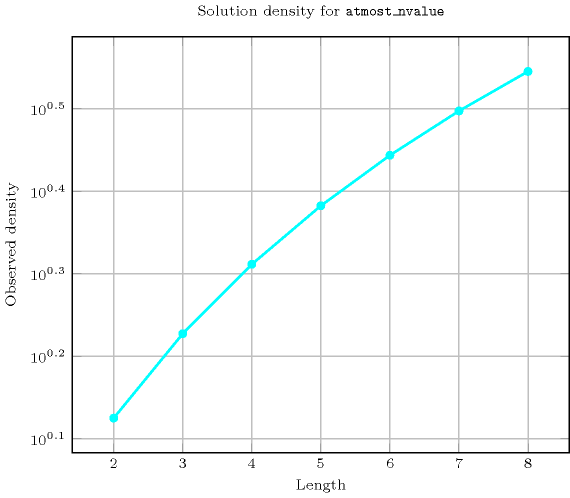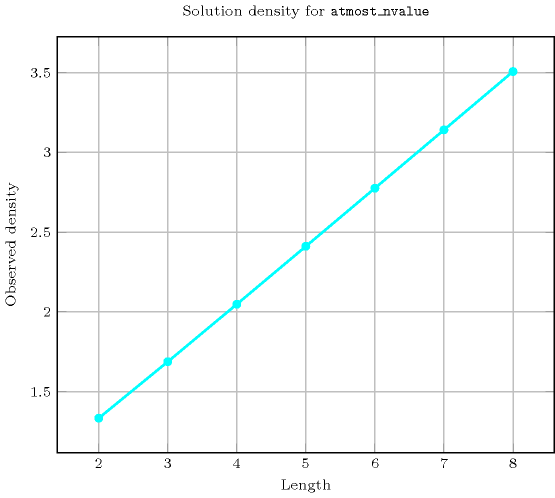Length ($n$)2345678
Total121081280187503265926588344150994944
 Parameter value

13456789
2940145456130935369153
3-64505345620209104672496017
4--6257056748096926725639841
5---7776112609163347221515841
6----117649205683237603521
7-----209715242683841
8------43046721

Solution count for $\mathrm{𝚊𝚝𝚖𝚘𝚜𝚝}_\mathrm{𝚗𝚟𝚊𝚕𝚞𝚎}$: domains $0..n$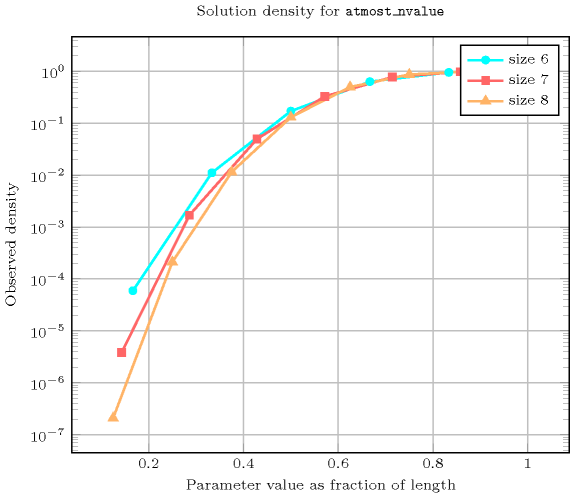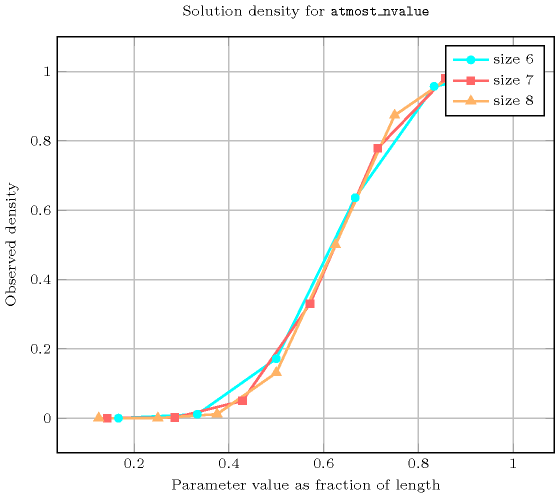Systems

implied by: $\mathrm{𝚗𝚟𝚊𝚕𝚞𝚎}$ ($\le$ $\mathrm{𝙽𝚅𝙰𝙻}$ replaced by $=$ $\mathrm{𝙽𝚅𝙰𝙻}$).

Keywords
Arc input(s)

$\mathrm{𝚅𝙰𝚁𝙸𝙰𝙱𝙻𝙴𝚂}$

Arc generator
$\mathrm{𝐶𝐿𝐼𝑄𝑈𝐸}$$↦\mathrm{𝚌𝚘𝚕𝚕𝚎𝚌𝚝𝚒𝚘𝚗}\left(\mathrm{𝚟𝚊𝚛𝚒𝚊𝚋𝚕𝚎𝚜}\mathtt{1},\mathrm{𝚟𝚊𝚛𝚒𝚊𝚋𝚕𝚎𝚜}\mathtt{2}\right)$

Arc arity
Arc constraint(s)
$\mathrm{𝚟𝚊𝚛𝚒𝚊𝚋𝚕𝚎𝚜}\mathtt{1}.\mathrm{𝚟𝚊𝚛}=\mathrm{𝚟𝚊𝚛𝚒𝚊𝚋𝚕𝚎𝚜}\mathtt{2}.\mathrm{𝚟𝚊𝚛}$
Graph property(ies)
$\mathrm{𝐍𝐒𝐂𝐂}$$\le \mathrm{𝙽𝚅𝙰𝙻}$

Graph class
$\mathrm{𝙴𝚀𝚄𝙸𝚅𝙰𝙻𝙴𝙽𝙲𝙴}$

Graph model

Parts (A) and (B) of Figure 5.41.1 respectively show the initial and final graph associated with the first example of the Example slot. Since we use the $\mathrm{𝐍𝐒𝐂𝐂}$ graph property we show the different strongly connected components of the final graph. Each strongly connected component corresponds to a specific value that is assigned to some variables of the $\mathrm{𝚅𝙰𝚁𝙸𝙰𝙱𝙻𝙴𝚂}$ collection. The 3 following values 1, 3 and 6 are used by the variables of the $\mathrm{𝚅𝙰𝚁𝙸𝙰𝙱𝙻𝙴𝚂}$ collection.

##### Figure 5.41.1. Initial and final graph of the $\mathrm{𝚊𝚝𝚖𝚘𝚜𝚝}_\mathrm{𝚗𝚟𝚊𝚕𝚞𝚎}$ constraint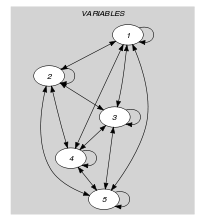(a)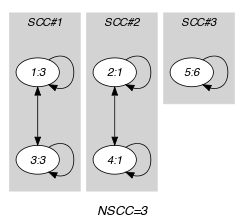(b)